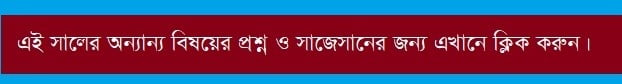# SSC All Board Mathematics Board Question 2019

SSC All Board Mathematics Board Question 2019. Mathematics is originated from the process of expressing quantities in symbols or numbers. The history of numbers is as ancient as the history of human civilization. Greek Philosopher Aristotle According to the formal inauguration of mathematics occurs in the practice of mathematics by the sect of the priest in ancient Egypt. So, the number based mathematics is the creation of about two thousand years before the birth of Christ. After that, moving from many nations and civilizations, numbers and principles of numbers have gained a universal form at present.

## SSC All Board Mathematics Board Question 2019SSC Mathematics Question 2019 Dhaka Board

SSC Mathematics Question 2019 Rajshahi Board

SSC Mathematics Question 2019 Dinajpur Board

SSC Mathematics Question 2019 Cumilla Board

SSC Mathematics Question 2019 Chittagong Board

SSC Mathematics Question 2019 Sylhet Board

SSC Mathematics Question 2019 Jashore Board

SSC Mathematics Question 2019 Barishal Board

Mathematics (from Greek μάθημα máthēma, “knowledge, study, learning”) includes the study of such topics as quantity (number theory), structure (algebra), space (geometry), and change (mathematical analysis). It has no generally accepted definition.

Mathematicians seek and use patterns to formulate new conjectures; they resolve the truth or falsity of conjectures by mathematical proof. When mathematical structures are good models of real phenomena, mathematical reasoning can be used to provide insight or predictions about nature. Through the use of abstraction and logic, mathematics developed from counting, calculation, measurement, and the systematic study of the shapes and motions of physical objects. Practical mathematics has been a human activity from as far back as written records exist. The research required to solve mathematical problems can take years or even centuries of sustained inquiry.

Rigorous arguments first appeared in Greek mathematics, most notably in Euclid’s Elements. Since the pioneering work of Giuseppe Peano (1858–1932), David Hilbert (1862–1943), and others on axiomatic systems in the late 19th century, it has become customary to view mathematical research as establishing the truth by rigorous deduction from appropriately chosen axioms and definitions. Mathematics developed at a relatively slow pace until the Renaissance when mathematical innovations interacting with new scientific discoveries led to a rapid increase in the rate of mathematical discovery that has continued to the present day.

Mathematics is essential in many fields, including natural science, engineering, medicine, finance, and the social sciences. Applied mathematics has led to entirely new mathematical disciplines, such as statistics and game theory. Mathematicians engage in pure mathematics (mathematics for its own sake) without having any application in mind, but practical applications for what began as pure mathematics are often discovered later.

During the Golden Age of Islam, especially during the 9th and 10th centuries, mathematics saw many important innovations building on Greek mathematics. The most notable achievement of Islamic mathematics was the development of algebra. Other notable achievements of the Islamic period are advances in spherical trigonometry and the addition of the decimal point to the Arabic numeral system. Many notable mathematicians from this period were Persian, such as Al-Khwarizmi, Omar Khayyam and Sharaf al-Dīn al-Ṭūsī.

During the early modern period, mathematics began to develop at an accelerating pace in Western Europe. The development of calculus by Newton and Leibniz in the 17th century revolutionized mathematics. Leonhard Euler was the most notable mathematician of the 18th century, contributing numerous theorems and discoveries. Perhaps the foremost mathematician of the 19th century was the German mathematician Carl Friedrich Gauss, who made numerous contributions to fields such as algebra, analysis, differential geometry, matrix theory, number theory, and statistics.

teachingbd24.com is such a website where you would get all kinds of necessary information regarding educational notes, suggestions and questions’ patterns of school, college, and madrasahs. Particularly you will get here special notes of physics that will be immensely useful to both students and teachers. The builder of the website is Mr. Md. Shah Jamal Who has been serving for 30 years as an Asst. Professor of BAF Shaheen College Dhaka. He expects that this website will meet up all the needs of Bengali version learners /students. He has requested concerned both students and teachers to spread this website home and abroad.